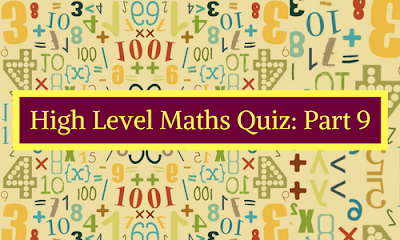Gurpurab Offer - Use Code GURPURAB2023

# High Level Maths Quiz: Part 9#### Question 1:

A man starts cycling at 12.00 a.m. at a speed of 5kmph. A train A , starts running on a track with a speed of 100kmph at 5.00 a.m. Another train B, starts running on the adjacent parallel track with a speed of 120 kmph at 7.00 am from the same station and in the same direction. Find the distance travelled by the man when the two trains meet.
a) 68km
b) 85km
c) 90 km
d) 92km

#### Question 2:

Pipe A can fill a cistern in 36 minutes and B in 48 minutes. If both the pipes are simultaneously opened together , when should pipe B be closed so that the cistern maybe just full in 24minutes ?
a) 8 mins
b)9mins
c)12mins
d)32 mins

#### Question 3:

How many words can be made by using the letters of the word FRIEND if all the vowels should be3 at even places and no letter is repeated or left ?
a)720
b)360
c)144
d) 48

Directions: Each of the questions below consists of a question and two statements numbered I and II given below it. You have to decide whether the data provided in the statements are sufficient to answer the question. Read both the statements and give answer-

a) If the data in both statements I and II together are not sufficient to answer the question.
b) If the data in both the statement I and II together are necessary to answer the question.
c) If either of the data in statement I alone or in statement II alone are sufficient to answer the question.
d) If the data in statement I alone are sufficient to answer the question, while the data in statement II alone are not sufficient to answer the question
e) If the data in statement II alone are sufficient to answer the question, while the data in statement I alone are not sufficient to answer the question

#### Question 4:

There are 240 students in a class some of who belong to the literary art club , the fine arts club or the debating group.It is given that one third of students do not belong toany of the clubs.If thereare 85 students who belong only to the fine arts club , how many students belong to the literary arts club ?

(i) There are 11 students who are in literary and fine arts but not in debating
(ii) There are 40 students in the debating club

#### Question 5:

What is the probability that an archer is able to hit a given target at a shooting range ?
(i) The probability that the archer hits a given target is 1/6 if the weather is clear
(ii) The probability that the weather is clear is 1/8

#### 1)

Let t be the time in hours after 5.00 am when the trains meet
Therefore distance covered
100 *t = 120 * (t-2)
T=12 hours
I.e. the trains meet at 5pm till then the man cycles for 17 hours
Distance covered by the man =5*17=85 km
b (85kms ) is the right answer

#### 2)

Let pipe B be turned off after x minutes
Part filled in x minutes = x ( 1/36 + 1/48) = 7x/144
Part filled in 24-x minutes = (24-x) / 36
7x/144 + 24-x/36 = 1
X=16 minutes , hence ( d) is the answer

#### 3)

We have six letters of which two are vowels. There are three even places where we can put two vowels in 3 * 2 ways = 6 ways
Remaining four places can be filled in 4*3*2*! = 24 ways
Hence, the number of words = 6*24 = 144 ways .Hence (c ) is the answer

#### 4)

(B) both the statements are together necessary to answer the question

#### 5)

The statement I or II individually do not give us any information needed, combining two we can get 1/6 * 1/8.However, we do not know what happens when the weather is not clear. Hence ( a) both statements combined also cannot answer